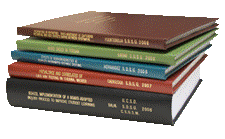## Libraries at University of Nebraska-Lincoln## Archival Master’s Theses from the University of Nebraska-Lincoln

#### Title

In Situ Measurement of Forage Yields

Thesis

6-1968

#### Citation

Thesis (M.S.)—University of Nebraska—Lincoln, 1968. Department of Agronomy.

Copyright 1968, the author. Used by permission.

#### Abstract

A review of the literature indicated that the electronic measurement of forage yields is an extremely promising method for the estimation of forage yields in situ. An instrument for estimating forage yields electronically was constructed at the University of Nebraska.

The objective of this study was to use the above electronic instrument to develop an equation for the prediction of forage yields and to test the equation under field conditions. In essence, the instrument is an electrical capacitance measuring unit in which the introduction of herbage to a measuring head causes a change in the electrical capacitance of the system. This capacitance change is measured on a meter and used as an indicator of the mass of herbage contained within the measuring head. The instrument is described and details are given of its use and operation.

In terms of a relationship between capacitance meter reading and forage yield as measured by the electronic probe and cutting, respectively, it was found that a significant linear relationship existed for each series of forage studied. However, significant differences existed between series predictive relationships.

The alfalfa prediction equation was relatively accurate in predicting harvested yields near the mean yield of the data used for the development of the equation. However, at higher yields the equation under-predicted the harvested yields and at lower yields the equation over-predicted the harvested yields. The inaccuracy of the prediction equation was due to the differences in moisture contents of the alfalfa that was used in developing the prediction equation. When variety yields were ranked, the predicted yields ranked relatively close to the harvested yields.

When trying to determine the value of the instrument, it should be emphasized that in its present form it would give estimate subject to considerable random error and to bias if it were used with only one prediction equation derived from pooled series data. But considering the good relationships which were established within forage species, it appears that the instrument could be used along with a regression derived for the specific species to obtain a relatively accurate estimate of plot yield.

The described instrument is potentially most valuable in the number of estimates which can be made. The estimates can be made at the rate of one every thirty seconds. Therefore, if the errors of prediction were entirely random errors, the greater efficiency of sampling by the instrument would compensate for any moderately increased error per prediction which it might have, compared with a less-efficient, more time-consuming cutting technique.

Since the taking of readings does very little damage to the pasture, the frequency of yield estimations may be increased to any number desired without causing much deviation from the normal growth of the sampled plot or pasture, as compared with the destroyed samples when a cutting technique is used.

The use of this electronic technique certainly appears promising and warrants further attention and research.